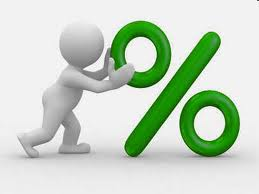# What is EMI and What are the smart ways to reduce loan interest?What is EMI and What are the smart ways to reduce loan interest?

If you take home loan or personal loan, you need to pay your monthly installment to a bank or financial institution. This is generally termed as EMI. What exactly does EMI mean? How you can calculate EMI of your loan amount? What are the various factors that would influence EMI of the loan? Are there any ways on how you can plan in smart ways and pay lesser interest on your EMI’s?

What is an EMI?

EMI means Equated Monthly Installments. This is the monthly repayment of the amount of loan taken by you. This loan could be a home loan, personal loan, consumer loan, the gold loan, etc. EMI has two components, one is principal and another is interest component.  Higher the principal, higher the interest amount you would pay and vice versa. In the initial period of payment of the loan, the interest payment is high and principal loan is very low. When the tenure goes on, interest reduces and your principal repayment would increase. EMI is adjusted in such a way that the interest amount is reduced month on month and principal repayment is increased.

Also Read: How to choose Best Home Loan in India?

How to calculate EMI for a loan amount?

EMI can be calculated with the below formula, though it is less complex.

EMI = P x R x (1+R)^n

——————–

(1+R)^n-1

P = Principal loan amount;  R = Rate of interest; N = Tenure of the loan in months

Explained with an example

• Mr.Akhil has taken Rs 25 Lakh home loan for a tenure of 20 years with LIC Housing Finance Ltd @ 10.2% interest rates.
• Here P = Principal Loan = Rs 25 Lakhs
• R = Rate of interest = 10.2% per annum and 0.85% per month
• N = Tenure of the loan = 20 x 12 = 240 months
• EMI works out to be Rs 24,458 per month
• Rs 25 Lakhs x 0.85% (1+0.85%)^240 / (1+0.85%)^240-1
• In excel, the formula would look like this =2500000*0. 85%* ((1+0.85%) ^240/((1+0.85%) ^240-1)

If you observe EMI value would fluctuate based on the principal amount and interest rate as majority.

What are the factors affecting an EMI?

EMI depends on 3 major factors. You can be smart enough to plan well and pay less interest.

1) Loan amount: This is the total loan taken by you. Highest the loan, the higher the EMI.

Suggestion: Prefer to take lowest loan if possible.

2) Interest Rate: Rate of interest is another major factor of EMI. Do you know that a small %age of higher interest rate, your EMI would zoom like anything.

Example-1- Rs 50 Lakhs home loan taken for a 20 year tenure.

• At 10.2% interest rate, monthly EMI works out to be Rs 48,915
• At 10.6% interest rate, monthly EMI works out to be Rs 50,255
• At 11% interest rate, monthly EMI works out to be Rs 51,609

Example-2- Rs 10 Lakhs personal loan taken for a 5 year tenure.

• At 12% interest rate, monthly EMI works out to be Rs 22,244
• At 14% interest rate, monthly EMI works out to be Rs 23,268
• At 16% interest rate, monthly EMI works out to be Rs 24,318

Suggestion: Prefer to take loans offering the lowest interest rates even if there is processing fees and complex procedure involved.

3) Tenure: Longer the tenure, interest would be grabbed by Banks in the first few years.

Suggestion: Do not close any short to medium term loans. You would have already paid the interest in the initial period and you need to pay a larger amount of principal payment when you want to repay at a later period of time. E.g. If you have taken 5 year person loan, majority of interest is already paid to bank in first 1-3 years. You should try to close such loans in early stage of the loan. Pre closure of such loan after this period, you would not benefit much.

Suresh
What is EMI and What are the smart ways to reduce loan interest

• Vehicle loans Auckland

EMI means Equated Monthly Installments. This is the monthly repayment of the amount of loan taken by you. This loan could be a home loan, personal loan, consumer loan, the gold loan, etc. EMI has two components, one is principal and another is interest component. Higher the principal, higher the interest amount you would pay and vice versa.Thanks for sharing it not just for me but for everyone.

• Sanjana

nice article. good information yaar

• Vinothkumar

I am owning 2 home loan , one is on my home town and another one is on City where i am working but still i changed my company so the house which i bought in city was  left unoccupied and i am residing in rented house , am i eligible to claim HRA as well ?

• Prasath

hi,
I tool home loan of 8L on 2009 for 10Y. can i close if now(July 2016). will i benefit?